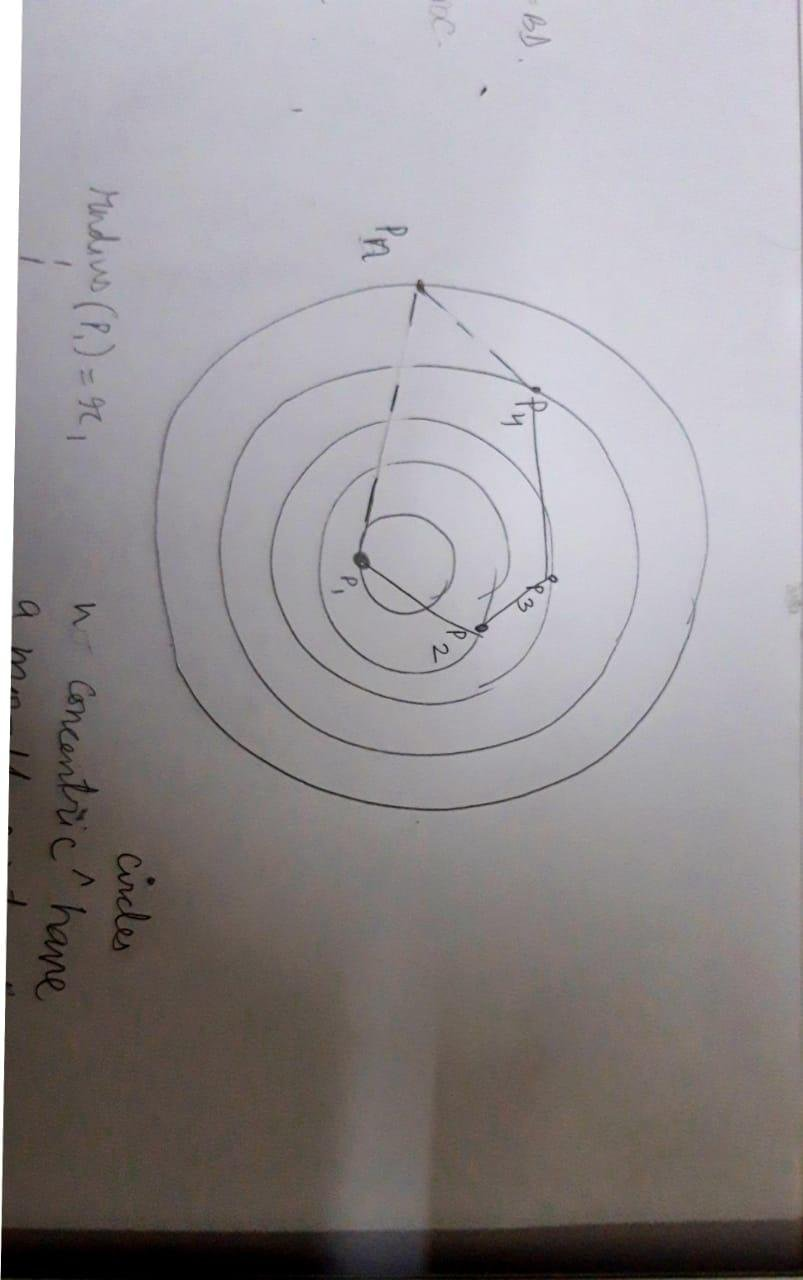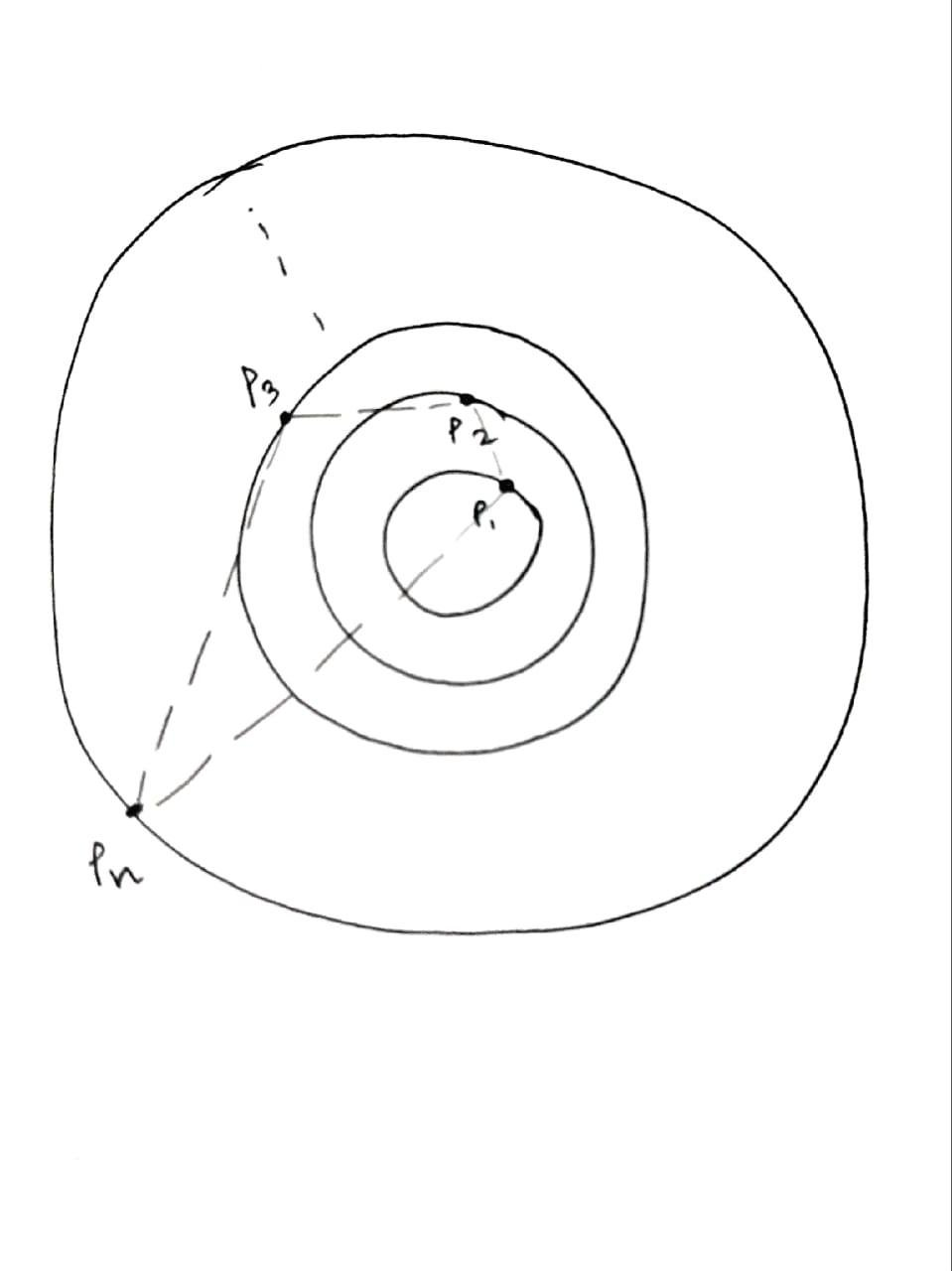# An open challenge

Home Forums Math Olympiad, I.S.I., C.M.I. Entrance An open challenge

Viewing 2 posts - 1 through 2 (of 2 total)
• Author
Posts
• #35575

This is a problem of my own volition:

given n concentric circles, each of which contain a point that can move on that circle, with radiu’s {r1, r2, …., rn}, find the geometric arrangement of these points to get a polygon of maximum area.

The attached figure will help elucidate the setup:#40907

Okay, so we are given a few concentric circles, we don’t know how many. I’ll give a few ideas of mine. Let me assume that they aren’t equidistant.

Plot the given figure in the Cartesian plane, with the common center as the origin (0,0).

[ A co-ordinate geometric approach]

Now we can fix a point on the first circle, then we can plot the other points variably, and then use the shoelace formula to find the co-ordinates as so to maximise the area.

Viewing 2 posts - 1 through 2 (of 2 total)
• You must be logged in to reply to this topic.